SBAS951B August   2019  – April 2020

PRODUCTION DATA.

1. Features
2. Applications
3. Description
1.     Device Images
4. Revision History
5. Pin Configuration and Functions
6. Specifications
7. Detailed Description
1. 7.1 Overview
2. 7.2 Functional Block Diagram
3. 7.3 Feature Description
4. 7.4 Device Functional Modes
8. Application and Implementation
1. 8.1 Application Information
2. 8.2 Typical Application
9. Power Supply Recommendations
10. 10Layout
11. 11Device and Documentation Support
1. 11.1 Device Support
1. 11.1.1 Device Nomenclature
2. 11.2 Documentation Support
4. 11.4 Community Resources
6. 11.6 Electrostatic Discharge Caution
7. 11.7 Glossary
12. 12Mechanical, Packaging, and Orderable Information

• DWV|8

### 6.2 Typical Characteristics

at AVDD = 5 V, DVDD = 3.3 V, AINP = –1 V to 1 V, AINN = AGND, fCLKIN = 20 MHz, and sinc3 filter with OSR = 256 (unless otherwise noted)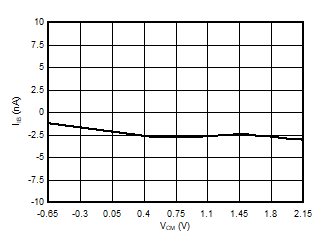Figure 6. Input Bias Current vs
Common-Mode Input Voltage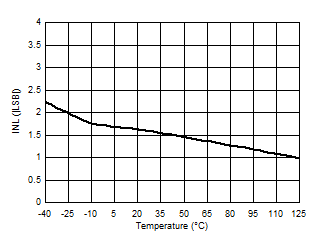Figure 8. Integral Nonlinearity vs Temperature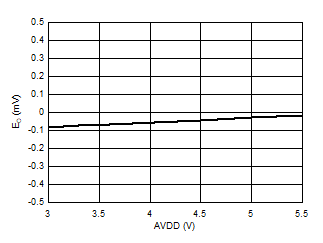Figure 10. Offset Error vs High-Side Supply Voltage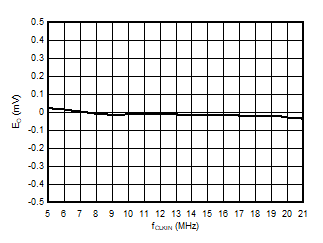fCLKIN < 9 MHz with AVDD ≥ 4.5 V only
Figure 12. Offset Error vs Clock Frequency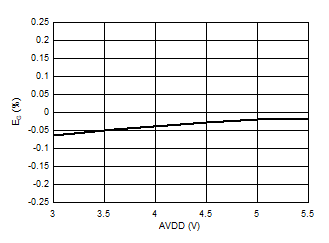Figure 14. Gain Error vs High-Side Supply Voltage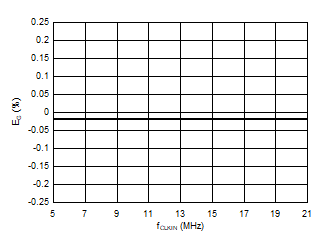fCLKIN < 9 MHz with AVDD ≥ 4.5 V only
Figure 16. Gain Error vs Clock Frequency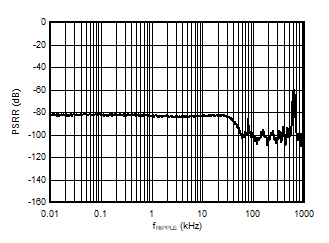Figure 18. Power-Supply Rejection Ratio vs
Ripple Frequency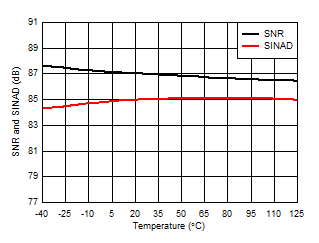Figure 20. Signal-to-Noise Ratio and Signal-to-Noise + Distortion vs Temperature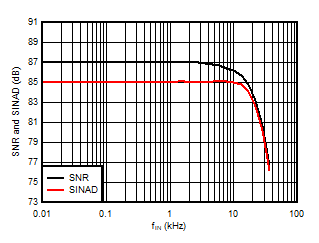Figure 22. Signal-to-Noise Ratio and Signal-to-Noise + Distortion vs Input Signal Frequency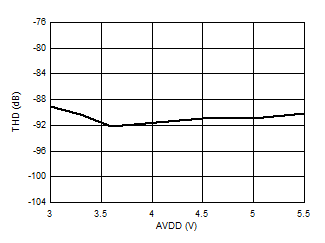Figure 24. Total Harmonic Distortion vs
High-Side Supply Voltage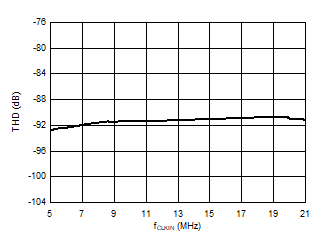fCLKIN < 9 MHz with AVDD ≥ 4.5 V only
Figure 26. Total Harmonic Distortion vs Clock Frequency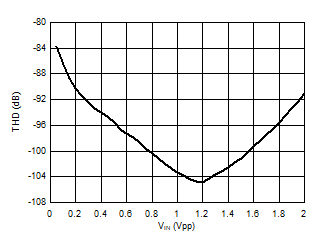Figure 28. Total Harmonic Distortion vs
Input Signal Amplitude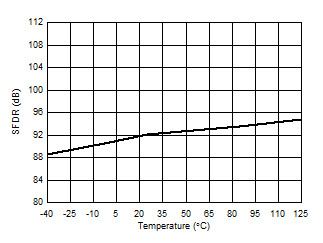Figure 30. Spurious-Free Dynamic Range vs Temperature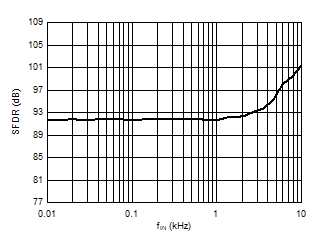Figure 32. Spurious-Free Dynamic Range vs
Input Signal Frequency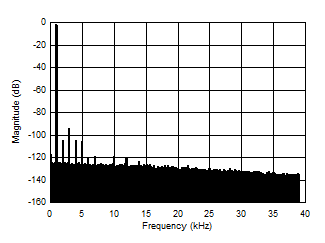156,250-point DFT, VIN = 2 VPP
Figure 34. Frequency Spectrum with 1-kHz Input Signal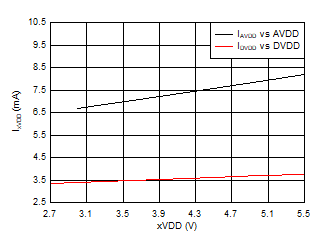Figure 36. Supply Current vs Supply Voltage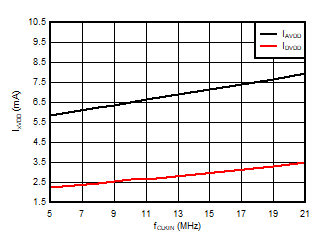fCLKIN < 9 MHz with AVDD ≥ 4.5 V only
Figure 38. Supply Current vs Clock Frequency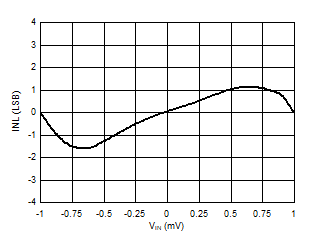Figure 7. Integral Nonlinearity vs
Input Voltage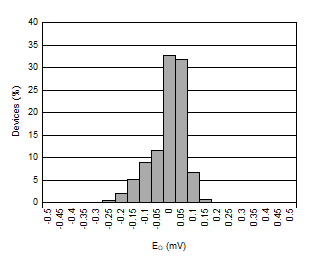Figure 9. Offset Error Histogram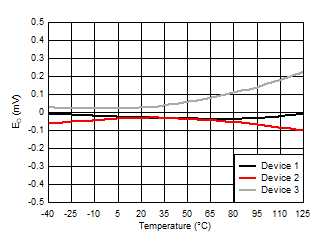Figure 11. Offset Error vs Temperature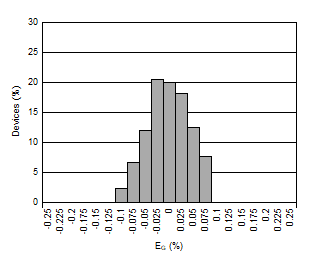Figure 13. Gain Error Histogram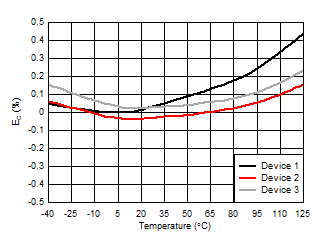Figure 15. Gain Error vs Temperature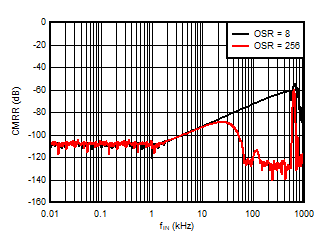Figure 17. Common-Mode Rejection Ratio vs
Input Signal Frequency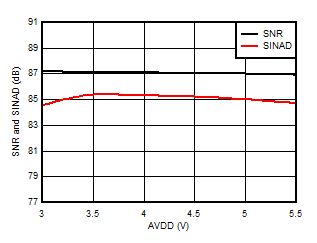Figure 19. Signal-to-Noise Ratio and Signal-to-Noise + Distortion vs High-Side Supply Voltage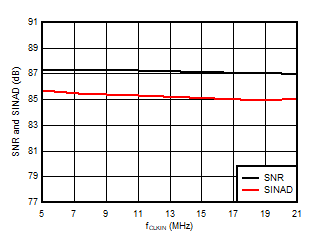fCLKIN < 9 MHz with AVDD ≥ 4.5 V only
Figure 21. Signal-to-Noise Ratio and Signal-to-Noise + Distortion vs Clock Frequency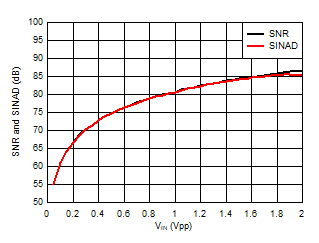Figure 23. Signal-to-Noise Ratio and Signal-to-Noise + Distortion vs Input Signal Amplitude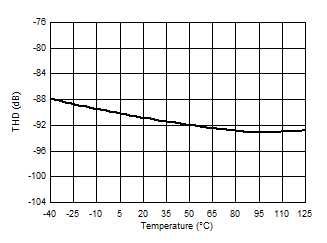Figure 25. Total Harmonic Distortion vs Temperature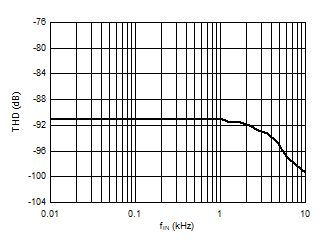Figure 27. Total Harmonic Distortion vs
Input Signal FrequencyFigure 29. Spurious-Free Dynamic Range vs
High-Side Supply Voltage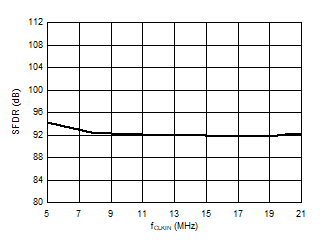fCLKIN < 9 MHz with AVDD ≥ 4.5 V only
Figure 31. Spurious-Free Dynamic Range vs
Clock Frequency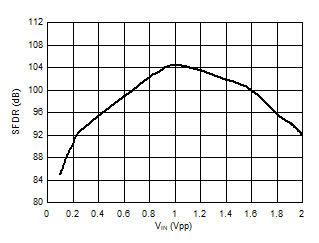Figure 33. Spurious-Free Dynamic Range vs
Input Signal Amplitude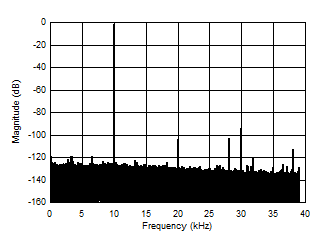156,250-point DFT, VIN = 2 VPP
Figure 35. Frequency Spectrum with 10-kHz Input Signal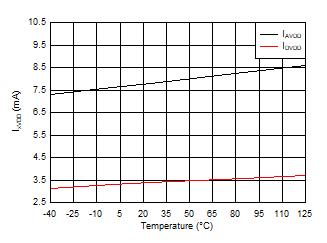Figure 37. Supply Current vs Temperature